Minima/ maxima (statement questions) - Geometry questions

Chapter 6 Class 12 Application of Derivatives
Concept wise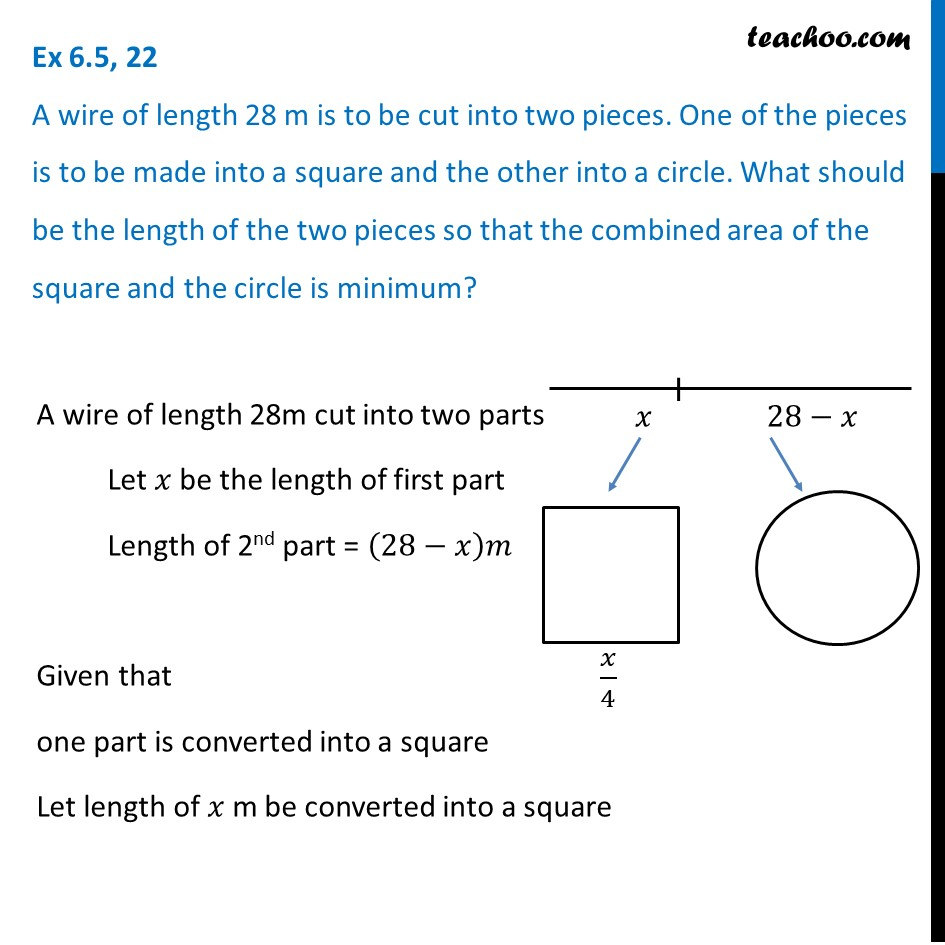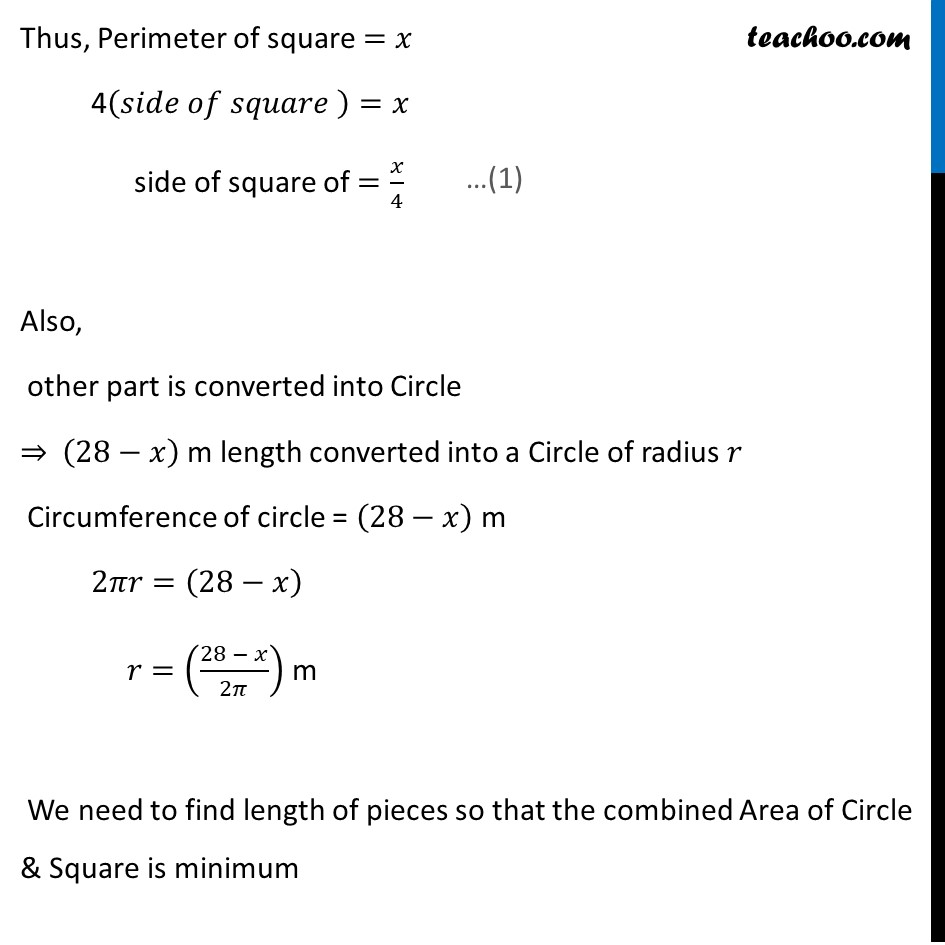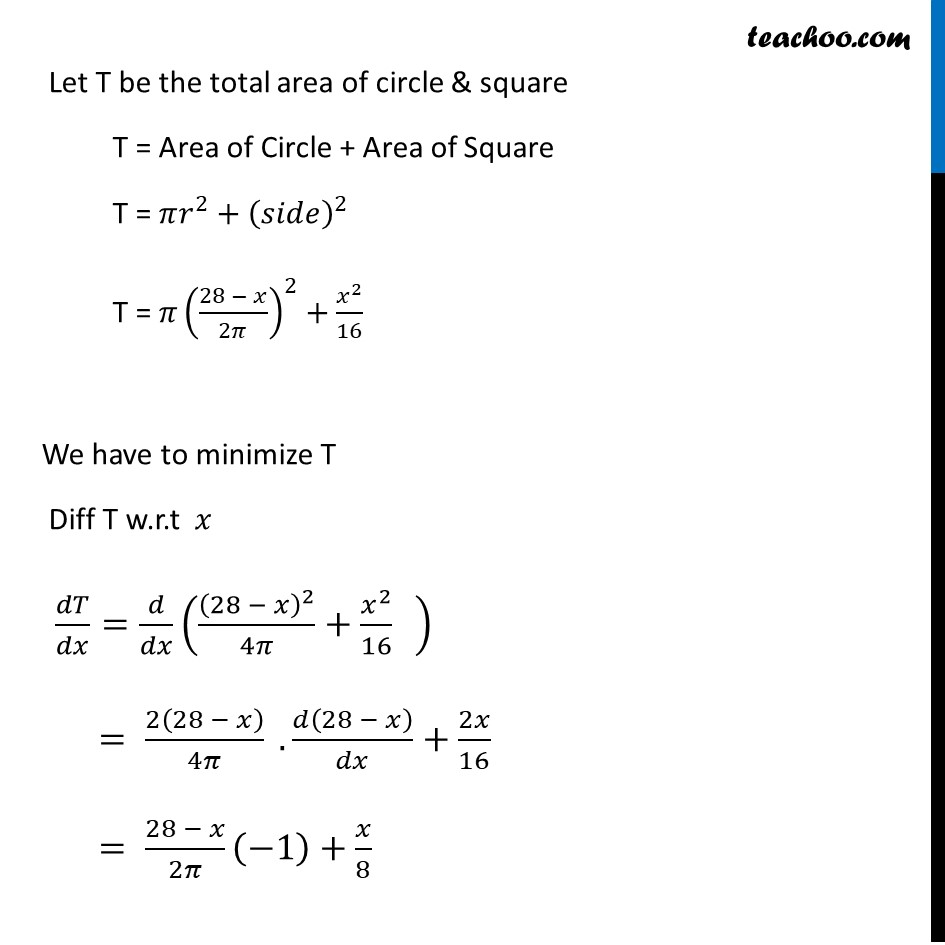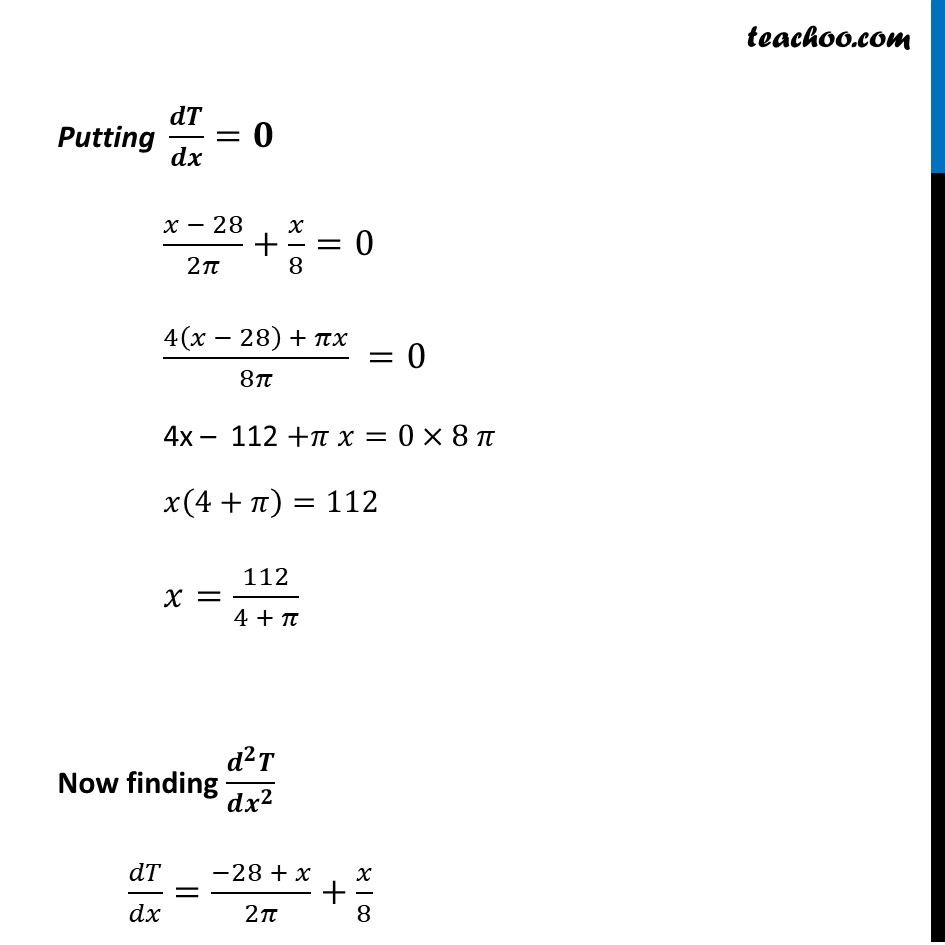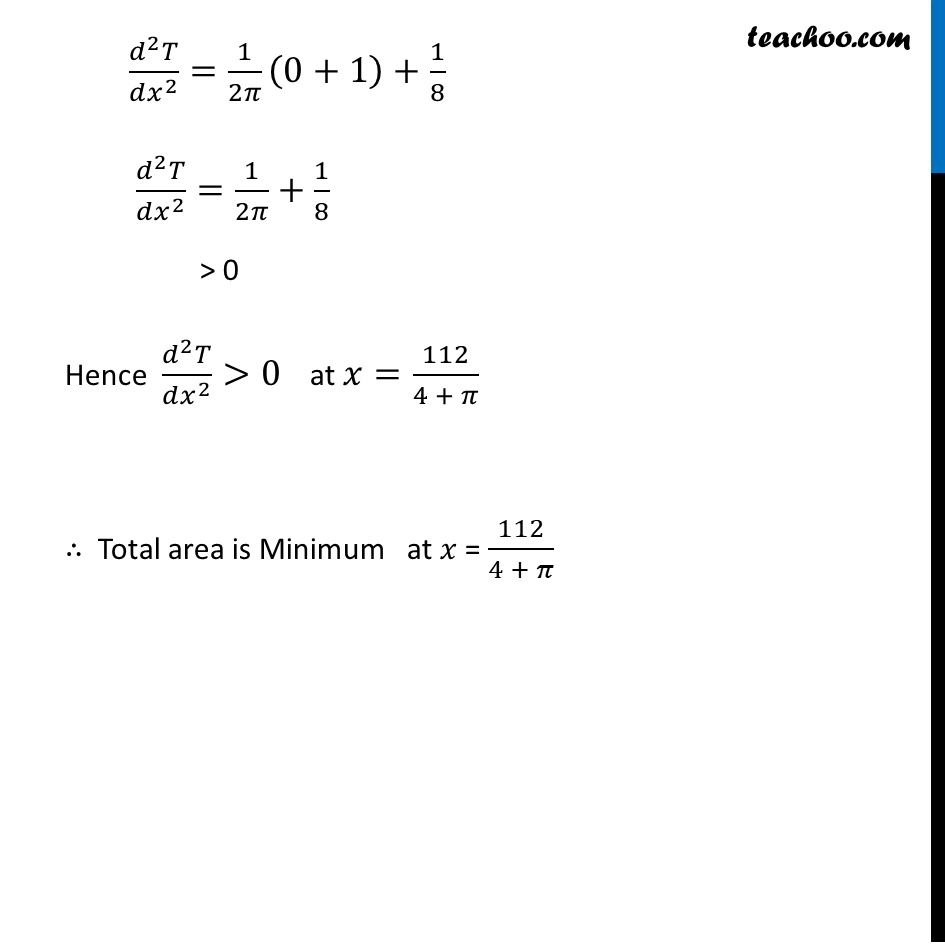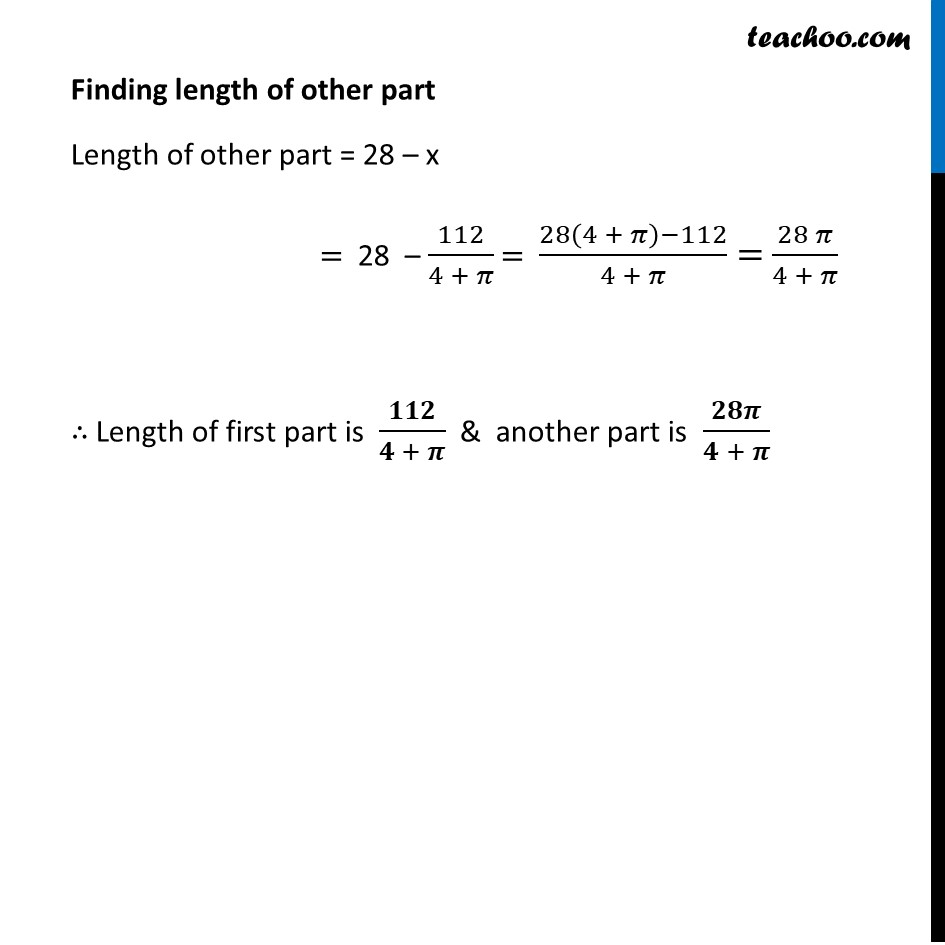Introducing your new favourite teacher - Teachoo Black, at only ₹83 per month

### Transcript

Ex 6.5, 22 A wire of length 28 m is to be cut into two pieces. One of the pieces is to be made into a square and the other into a circle. What should be the length of the two pieces so that the combined area of the square and the circle is minimum? A wire of length 28m cut into two parts Let 𝑥 be the length of first part Length of 2nd part = (28−𝑥)𝑚 Given that one part is converted into a square Let length of 𝑥 m be converted into a square Thus, Perimeter of square =𝑥 4(𝑠𝑖𝑑𝑒 𝑜𝑓 𝑠𝑞𝑢𝑎𝑟𝑒 )=𝑥 side of square of =𝑥/4 Also, other part is converted into Circle ⇒ (28−𝑥) m length converted into a Circle of radius 𝑟 Circumference of circle = (28−𝑥) m 2𝜋𝑟=(28−𝑥) 𝑟=((28 − 𝑥)/2𝜋) m We need to find length of pieces so that the combined Area of Circle & Square is minimum Let T be the total area of circle & square T = Area of Circle + Area of Square T = 𝜋𝑟^2+(𝑠𝑖𝑑𝑒)^2 T = 𝜋((28 − 𝑥)/2𝜋)^2+𝑥^2/16 We have to minimize T Diff T w.r.t 𝑥 𝑑𝑇/𝑑𝑥=𝑑/𝑑𝑥 ((28 − 𝑥)^2/4𝜋+𝑥^2/16 " " ) = 2(28 − 𝑥)/4𝜋 . 𝑑(28 − 𝑥)/𝑑𝑥+2𝑥/16 = (28 − 𝑥)/2𝜋 (−1)+𝑥/8 Putting 𝒅𝑻/𝒅𝒙=𝟎 (𝑥 − 28)/2𝜋+𝑥/8=0 (4(𝑥 − 28) + 𝜋𝑥)/8𝜋 =0 4x – 112 +𝜋 𝑥=0×8 𝜋 𝑥(4+𝜋)=112 𝑥=112/(4 + 𝜋) Now finding (𝒅^𝟐 𝑻)/(𝒅𝒙^𝟐 ) 𝑑𝑇/𝑑𝑥=(−28 + 𝑥)/2𝜋+𝑥/8 (𝑑^2 𝑇)/(𝑑𝑥^2 )=1/2𝜋 (0+1)+1/8 (𝑑^2 𝑇)/(𝑑𝑥^2 )=1/2𝜋+1/8 > 0 Hence (𝑑^2 𝑇)/(𝑑𝑥^2 )>0 at 𝑥=112/(4 + 𝜋) ∴ Total area is Minimum at 𝑥 = 112/(4 + 𝜋) Finding length of other part Length of other part = 28 – x = 28 – 112/(4 + 𝜋) = (28(4 + 𝜋)−112)/(4 + 𝜋)= (28 𝜋)/(4 + 𝜋) ∴ Length of first part is 𝟏𝟏𝟐/(𝟒 + 𝝅) & another part is 𝟐𝟖𝝅/(𝟒 + 𝝅)